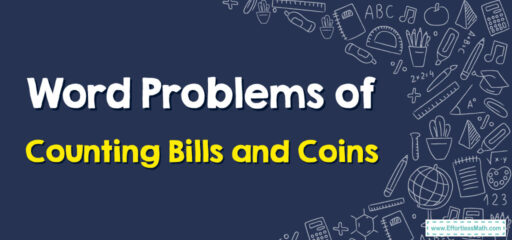# How to Solve Word Problems of Counting Bills and Coins

Word problems involving counting bills and coins require understanding the value of each type of bill and coin, and then adding those values together.## A Step-by-step Guide to Solving Word Problems of Counting Bills and Coins

Here’s a simple step-by-step guide for students to solve word problems involving counting bills and coins:

### Step 1: Understand the Problem

First, read the problem carefully. Understand what it’s asking you. It could be asking how much money someone has in total or how much more money they need to buy something.

### Step 2: Identify the Bills and Coins

Write down the number of each bill and coin mentioned in the problem. Remember the value of each:

• A penny is worth 1 cent ($0.01) • A nickel is worth 5 cents ($0.05)
• A dime is worth 10 cents ($0.10) • A quarter is worth 25 cents ($0.25)
• A one-dollar bill is worth 1 dollar ($1.00) • A five-dollar bill is worth 5 dollars ($5.00)
• A ten-dollar bill is worth 10 dollars ($10.00) ### Step 3: Multiply and Add Multiply the value of each bill or coin by the number of them you have. Then add all these values together to find the total amount of money. ### Step 4: Answer the Question Use the total amount of money to answer the question. ## Related to This Article ### More math articles ### What people say about "How to Solve Word Problems of Counting Bills and Coins - Effortless Math: We Help Students Learn to LOVE Mathematics"? No one replied yet. X 30% OFF Limited time only! Save Over 30% SAVE$5

It was $16.99 now it is$11.99